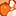# QlikView App Dev

Discussion Board for collaboration related to QlikView App Development.

Announcements
Our next Qlik Insider session will cover new key capabilities. Join us August 11th REGISTER TODAY
cancel
Showing results for
Did you mean:Creator II

## Expressions

Hi,

I have 2 expressions and i made modifications to them. So my question is, are the changes done by me working fine or not ?

I mean the new expressions will be giving the same outputs as old expressions outputs or not.

1. OLD_Expr:

sum(IF(Guidance_Method_for_APT='MBG',If(SUM(TARGET_REVENUE)=0,0,SUM(NET_REVENUE)),

IF(Guidance_Method_for_APT='DBG',sum(NET_REV_GUID_DBG))))

NEW_Expr:

SUM({<TARGET_REVENUE-={0},Guidance_Method_for_APT={'MBG'}>}NET_REVENUE)

+

sum({<Guidance_Method_for_APT={'DBG'}>}NET_REV_GUID_DBG)

2.OLD_Expr:

SUM(IF(Guidance_Method_for_APT='MBG',If(SUM(TARGET_REVENUE)=0,0,SUM(NET_REVENUE)),IF(Guidance_Method_for_APT='DBG',sum(NET_REV_GUID_DBG))))

/

SUM(IF(Guidance_Method_for_APT='MBG',SUM(TARGET_REVENUE),IF(Guidance_Method_for_APT='DBG',sum(TARGET_REVENUE_DBG))))

NEW_Expr:

(SUM({<TARGET_REVENUE-={0},Guidance_Method_for_APT={'MBG'}>}NET_REVENUE)

+

sum({<Guidance_Method_for_APT={'DBG'}>}NET_REV_GUID_DBG))

/

(sum({<Guidance_Method_for_APT={'MBG'}>}TARGET_REVENUE)

+

sum({<Guidance_Method_for_APT={'DBG'}>}TARGET_REVENUE_DBG))

Please check and let me know.

Thank you

7 RepliesContributor III

Hi ,

Can you share Sample Files.Creator III

Hi,

Share some sample data so we can use your expression for verification.

Thanks,Creator III

Hi,

From first view ...it seems that your results will be different as you are adding up data in set analysis while in if condition you have given on addition in else statement.

Share your sample data for more clarity.

Thanks,Creator II
AuthorCreator II
AuthorCreator III

Hi Mohan,

Here scenario is different beacuse we are comparing with your old and new expressions.means if and ste analysis conditions.

Thanks,MVP

Do you check values for same, If so that would be correct. If not, it won't be correct values please check and let us know so that we can move next steo

Before develop something, think If placed (The Right information | To the right people | At the Right time | In the Right place | With the Right context)Community Browser